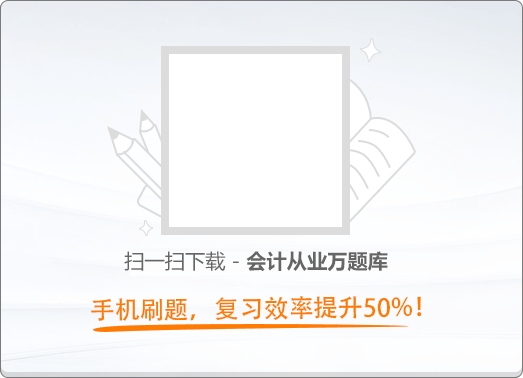60:00《会计电算化》2016新大纲真题精编（八）

1
(单项选择题)

• A.

成本高

• B.

见效慢

• C.

维护没有保障

• D.

软件针对性不强

• A
• B
• C
• D

2
(单项选择题)

• A.

成本管理模块

• B.

财务分析模块

• C.

账务处理模块

• D.

工资管理模块

• A
• B
• C
• D

3
(单项选择题)

• A.

人机结合

• B.

会计核算自动化、集中化

• C.

数据处理及时准确

• D.

内部控制比较单一

• A
• B
• C
• D

4
(单项选择题)

• A.

系统初始化设置

• B.

数据输入

• C.

期末处理

• D.

日常处理

• A
• B
• C
• D

5
(单项选择题)

• A.

将该U盘报废

• B.

换一台计算机继续使用该U盘

• C.

将该U盘文件复制到另一个U盘上使用

• D.

用杀毒软件清除该U盘上的病毒或在确认无病毒的计算机上格式化该U盘

• A
• B
• C
• D

6
(单项选择题)

• A.

Sybase

• B.

UNIX

• C.

FoxPro

• D.

SQL Server

• A
• B
• C
• D

7
(单项选择题)

• A.

初始单据

• B.

初始票据

• C.

初始坏账

• D.

初始现金

• A
• B
• C
• D

8
(单项选择题)

• A.

数据的筛选是指利用“数据”菜单中的“筛选”命令对数据清单中的指定数据进行查找和其他工作

• B.

筛选后的数据清单仅显示那些包含了某一特定值或符合一组条件的行，暂时隐藏其他行

• C.

通过筛选的工作表中的信息，用户可以快速查找数据

• D.

在进行数据筛选时，用户只可以利用筛选功能控制需要显示的内容，不能够控制需要排除的内容

• A
• B
• C
• D

9
(单项选择题)

• A.

选定某单元格时，可用鼠标指针指向它，单击鼠标左键即可

• B.

被选定的单元格称活动单元格

• C.

不允许一次选定多个单元格

• D.

当前工作表中的单元格只有唯一的列标、行号与之对应

• A
• B
• C
• D

10
(单项选择题)

• A.

创建的图表只能放在含有用于创建图表数据的工作表之中

• B.

图表建立之后，不允许再向图表中添加数据，若要添加只能重新建立

• C.

图表建立后，可改变其大小、移动、删除等，但不能改变其类型，如柱形图改为饼形图

• D.

若要修饰图表，必须先选定图表，将其激活

• A
• B
• C
• D